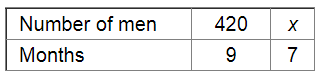# A work-force of 420 men with a contractor can finish a certain piece of work in 9 months.`
Question:

A work-force of 420 men with a contractor can finish a certain piece of work in 9 months. How many extra men must he employ to complete the job in 7 months?

Solution:

Let x be the extra number of men employed to complete the job in 7 months.Since the number of men hired and the time required to finish the piece of work are in inverse variation, we have :

$420 \times 9=7 x$

$\Rightarrow x=\frac{420 \times 9}{7}$

$=540$

Thus, the number of extra men required to complete the job in 7 months $=540-420=120$